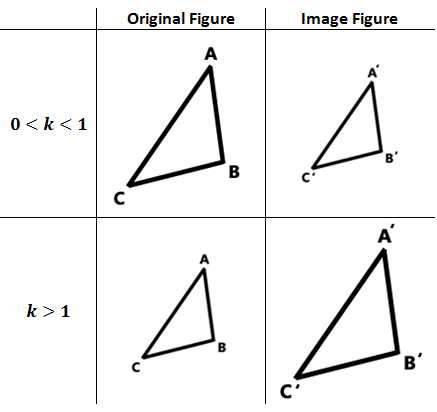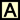Dilations
 Home > Lessons > Dilations Search | Updated August 5th, 2018
Introduction
In this section of MATHguide, you will learn about dilations. Here are the topics within this page:

 Characteristics Similar Figures -- a topic covered in another section -- can be described by the mathematics of dilations.     Dilations have these features: They have a center of dilation. They have a scale factor. There is the original figure and it's image figure. Labeling Dilations When we refer to the original figure, of say a triangle, we call the original figure △ABC. We call the image figure △A'B'C'. The second triangle is pronounced, "triangle A-prime, B-prime, C-prime." Scale Factor A characteristic called scale factor informs us of the relationship between the original figure and its image. The scale factor is typically called the k-value.     When the k-value is 0 < k < 1 [greater than zero but less than one], the image figure is smaller than the original figure. When the k-value is k > 1 [greater than one], the image figure is greater than the original figure. This information is encapsulated within the diagram that follows.Instructional Videos The video below will demonstrate exactly how dilations work.ideo: Dilations Interactive Quizzes Here is a quiz that can be used to test your understanding of dilations.uiz: Dilations Activities This is a lesson that uses scale factors. After this lesson, students will be ready to move on to Areas and Lengths of Similar Figures and Volumes and Lengths of Similar Solids (see the related lessons section below).ctivity: Scale Model of the Solar Systemctivity: Planet Informationctivity: Scale Solar System (Excel) Related Lessons View these related lessons.esson: Reducing Fractionsesson: Geometry Proofsesson: Proportionsesson: Similar Figuresesson: Areas and Lengths of Similar Figuresesson: Volumes and Lengths of Similar Solids